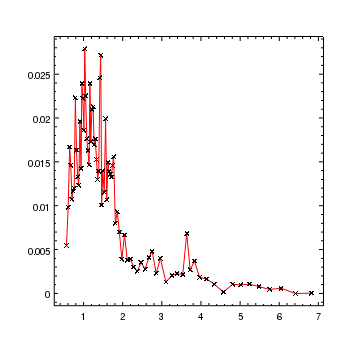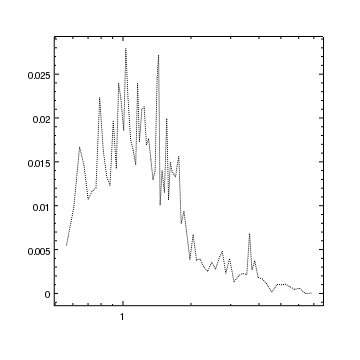Skip to the navigation links

# Gallery: Basic curves

## 1) A curve marked with symbols and connected by a red line

Curves are used to plot (x, y) data, with optional error bars on both axes. A later example shows how to display error bars on the points.Version: Postscript; PDF
```add_curve("spectrum.fits[cols x,y]",["symbol.size",3,"line.color","red"])
```

The default appearance of curves is to display each point as a cross, connected by a solid line. The symbol style and line style can be changed, but in this plot we just override the default symbol size and line color when the curve is created.

The preferences for curves can be found by using the get_preference call:

```chips> print(get_preference("curve"))
curve.stem             : crv
curve.baddata          : omit
curve.depth            : default
curve.line.color       : default
curve.line.thickness   : 1
curve.line.style       : solid
curve.symbol.color     : default
curve.symbol.style     : cross
curve.symbol.size      : 5
curve.symbol.angle     : 0
curve.symbol.fill      : true
curve.err.color        : default
curve.err.thickness    : 1
curve.err.style        : line
curve.err.up           : on
curve.err.down         : on
curve.err.left         : on
curve.err.right        : on
curve.err.caplength    : 10
curve.limit.length     : 0.05
curve.limit.override   : true

```

The settings for the current curve can be found by using the get_curve routine:

```chips> get_curve()

baddata = 1
depth = 100
err.caplength = 10
err.color = default
err.down = False
err.left = False
err.right = False
err.style = line
err.thickness = 1.0
err.up = False
id = None
limit.length = 0.0500000007451
limit.override = True
line.color = red
line.style = 1
line.thickness = 1.0
stem = None
symbol.angle = 0.0
symbol.color = default
symbol.fill = True
symbol.size = 3
symbol.style = 1

```

## 2) A curve marked with diamondsVersion: Postscript; PDF
```crv = ChipsCurve()
crv.line.style = "noline"
crv.symbol.style = "diamond"
crv.symbol.size = 3
add_curve("spectrum.fits[cols x,y]",crv)
log_scale(Y_AXIS)
```

In this plot we hide the line connecting the points by setting its style to noline and change the symbol to diamond. After the plot has been created the Y axis is changed to use a logarithmic scale using the log_scale command.

## 3) A curve connected by a dotted lineVersion: Postscript; PDF
```crv = ChipsCurve()
crv.line.style = "dot"
crv.symbol.style = "none"
add_curve("spectrum.fits[cols x,y]",crv)
log_scale(X_AXIS)
```

Curves can also be displayed as a set of connected lines with no symbols, as in this example. The line can be drawn in one of a number of styles; this example uses the dotted version.

## 4) A curve showing the full range of options

This example shows some of the flexibility of curves by changing how the symbol, line, and error bars are drawn. It also illustrates that curves can be created from arrays, not just read in from data files.Version: Postscript; PDF
```# Create x, y, and error arrays
x = np.arange(1,10,2)
y = x**2
dx = x*0 + 1
dylo = y*0.15
dyhi = y*0.1
add_curve(x,y,[dylo,dyhi,dx,dx])

# Change the curve properties
crv = ChipsCurve()
crv.line.color = "red"
crv.line.style = "longdash"
crv.symbol.color = "green"
crv.symbol.style = "circle"
crv.err.style = "capped"
crv.err.color = "blue"
set_curve(crv)

log_scale(Y_AXIS)
set_plot_title("y=x^2")
```

The first set of lines create a set of (x,y) points with asymmetric error bars. For the purposes of this example plot simple numerical relations are used. Error values are indicated by the third argument being a list of arrays, where the number of elements of this list determine how the arrays are used, as given in the table below. All error values are relative and should be positive.

Error array Meaning
[dy] Symmetric y errors
[dyl, dyh] Asymmetric y errors (low values then high values)
[dyl, dyh, dxl, dxh] Asymmetric y and then x errors (low values then high values)

A ChipsCurve object is used to change the curve attributes; in this case the choices are made to highlight some of the control that is provided by ChIPS. The set_curve command is used to apply these new values.

Note that the Y errors are displayed correctly even when the axis has been changed to use a logarithmic scale.